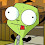## Thursday, April 22, 2010

### first loop in the vm!

I've implemented enough instructions in the VM that I can now do a loop (a.k.a. a tail-recursive function call).

I still need to work on the VM insns, there's a lot of allocation taking place that shouldn't... also, there's range-checking on each and every vector access.. even including stuff like the register file. Given those caveats, it's only about 4X slower than a similar python loop. I'm pretty sure I should be able to make it faster than python, we'll see.

```--- cps ---
0 = new_env [] 1
-   push_env  None
1 = close [] 'loop_0'
0 = lit [] 3
1 = varref [] ((0, 0), False, {n_1})
0 = primop [0, 1] ('%=',)
0 = test  None
0 = lit [] 1
return  None
0 = varref [] ((0, 0), False, {n_1})
1 = lit [] 2
0 = primop [0, 1] ('%-',)
tr_call  (1, )
return  None
-   store_tuple [1, 0] (0, 1, 1)
0 = new_env [] 1
1 = lit [] 0
0 = store_tuple [1, 0] (0, 1, 1)
1 = varref [] ((0, 0), True, {loop_0})
invoke_tail [1, 0]
```

1.2.